## Sunday, October 21, 2007

### Irodov Problem 1.88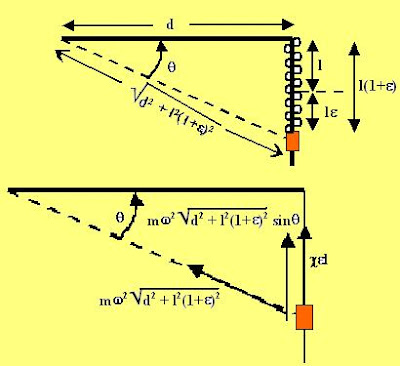Let the original length of the spring be l and suppose that because of the rotation the spring elongates by a length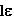(is the percentage elongation of the spring). Thus, the total length of the spring will be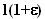. The angleformed by the mass to the pivot (as shown int the figure) is given by,From the point of view of a stationary observer there is only one force acting on the mass - the force of the spring that tries to pull the mass back given by. The mass because of its rotation is subject to a centripetal acceleration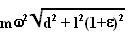that is directed radially inwards towards the pivot (this will become centrifugal force directed outwards with respect to a rotating observer on the mass). The component of this centripetal acceleration along the direction of the spring is given by,Now writing the F=ma due to Newton we have,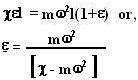No matter what the direction of rotation the centripetal acceleration will always be directed in the same direction and will have the same magnitude. In other words, the rotation direction will not matter - the elongation will be the same.